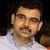# App Development

Announcements
Join “Do More With Qlik” with Mike Tarallo to get a first-hand look at the New Authoring Experience, June 29th: REGISTER HERE
cancel
Showing results for
Did you mean:Not applicable

## Reutilize master measure in another master measure in Qlik Sense

I've noticed that now you can use master measure in another master measure, eg.:

Meas. 1               TotalAmount              =       Sum(Amount)

Meas. 2               TotalQty                    =        Sum(Qty)

Meas. 3                Average                    =       TotalAmount/TotalQty

If you put in a table all the three master measures they work fine, but if you remove Meas1 or Meas2 you have null in the Meas3 column.

The same apply if you use Meas3 alone.

Anyone has solved the issue?

1 Solution

Accepted SolutionsMVP

You can't use master measures as if they are variables. The measures only make sense in the context of the object they're used. What you want instead is variables and use those. In Qlik Sense 2.1 you can create variables in the user interface. In older versions you need to create them in the script.

SET vTotalAmount = sum(Amount);

SET vTotalQty = sum(Qty);

LET vAverage = '\$('& 'vTotalAmount)/\$(' & 'vTotalQty)';

You can then use the variables in the measures:

=\$(vTotalAmount)

=\$(vTotalQty)

=\$(vAverage)

talk is cheap, supply exceeds demand
5 RepliesPartner

Hi,

you can can move measure definitions to variables:

Meas. 1   TotalAmount = \$(vTotalAmount)

Meas. 2   TotalQty = \$(vTotalQty)

Meas. 3   Average = \$(vAverage)

Variables:

vTotalAmount = Sum(Amount)

vTotalQty= Sum(Qty)

vAverage = \$(vTotalAmount)/\$(vTotalQty)MVP

You can't use master measures as if they are variables. The measures only make sense in the context of the object they're used. What you want instead is variables and use those. In Qlik Sense 2.1 you can create variables in the user interface. In older versions you need to create them in the script.

SET vTotalAmount = sum(Amount);

SET vTotalQty = sum(Qty);

LET vAverage = '\$('& 'vTotalAmount)/\$(' & 'vTotalQty)';

You can then use the variables in the measures:

=\$(vTotalAmount)

=\$(vTotalQty)

=\$(vAverage)

talk is cheap, supply exceeds demandNot applicable
Author

Anyway you (and Alex) are right.MVP

You can still use the Master Items. You just have to create them using the variables.

talk is cheap, supply exceeds demandLuminary Alumni

I created a short video on how to re-use master items.

https://youtu.be/YZ-bc5Sx_Bk